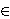### Ke Gong

Department of Mathematics, Tongji University, Shanghai 200092, P. R. China
e-mail: kogong@gmail.com

Abstract.   We give nontrivial bounds in various ranges for character sums of the form

nS(x,y) χ(R1(n))eq(R2(n)),

where χ is a nonprincipal multiplicative character modulo a prime q, R1 and R2 are rational functions modulo q, and S(x,y) is the set of positive integers nx that are divisible only by primes py. We also give sharper bounds in some special cases.

2000 Mathematics Subject Classification.   11L40, 11L20, 11N25.

Key words and phrases.   Character sums, rational functions, smooth numbers.

Full text (PDF) (free access)

DOI: 10.3336/gm.44.2.06

References:

1. J. Bourgain, More on the sum-product phenomenon in prime fields and its applications, Int. J. Number Theory 1 (2005), 1-32.
MathSciNet     CrossRef

2. R. de la Bretèche, G. Tenenbaum, Sommes d'exponentielles friables d'arguments rationnels, Funct. Approx. Comment. Math. 37 (2007), 31-38.
MathSciNet

3. É. Fouvry, P. Michel, Sur certaines sommes d'exponentielles sur les nombres premiers, Ann. Sci. École Norm. Sup. (4) 31 (1998), 93-130.
MathSciNet     CrossRef

4. É. Fouvry, G. Tenenbaum, Entiers sans grand facteur premier en progressions arithmetiques, Proc. London Math. Soc. (3) 63 (1991), 449-494.
MathSciNet     CrossRef

5. H. Iwaniec, E. Kowalski, Analytic Number Theory, American Mathematical Society, Providence, RI, 2004.
MathSciNet

6. A. A. Karatsuba, Sums of characters with prime numbers, Math. USSR Izv. 4 (1970), 303-326.
CrossRef

7. A. A. Karatsuba, Sums of Legendre symbols of polynomials of second degree over prime numbers, Math. USSR Izv. 12 (1978), 299-308.
CrossRef

8. A. A. Karatsuba, Arithmetic problems in the theory of Dirichlet characters, Russ. Math. Surv. 63 (2008), 641-690.
MathSciNet     CrossRef

9. A. A. Kopaneva, Application of Postnikov's formula for estimation of short sums of Dirichlet characters over shifted prime numbers, Moscow Univ. Math. Bull. 63 (2008), 218-220.
CrossRef

10. H. Maier, Exponential sums with multiplicative coefficients over smooth integers, Funct. Approx. Comment. Math. 35 (2006), 209-218.
MathSciNet

11. G. I. Perel'muter, On some sums with characters, Uspekhi Mat. Nauk 18 (1963), 145-149. (Russian)

12. G. I. Perel'muter, Evaluation of a sum containing primes, Soviet Math. Dokl. 3 (1962), 663-667.

13. Z. Kh. Rakhmonov, On the distribution of values of Dirichlet characters and their applications, Proc. Steklov Inst. Math. 207 (1995), 263-272.
MathSciNet

14. I. E. Shparlinski, Character sums over shifted smooth numbers, Proc. Amer. Math. Soc. 135 (2007), 2699-2705.
MathSciNet     CrossRef

15. R. C. Vaughan, A new iterative method in Waring's problem, Acta Math. 162 (1989), 1-71.
MathSciNet     CrossRef